Courses

# Integration in economics and commerce - Integration, Business Mathematics & Statistics B Com Notes | EduRev

## B Com : Integration in economics and commerce - Integration, Business Mathematics & Statistics B Com Notes | EduRev

The document Integration in economics and commerce - Integration, Business Mathematics & Statistics B Com Notes | EduRev is a part of the B Com Course Business Mathematics and Statistics.
All you need of B Com at this link: B Com

The cost function and average cost function from marginal cost function : If C is the cost of producing an output x then marginal cost dC function, mc = dc/dx Using integration as reverse process of differentiation we obtain,

Cost function,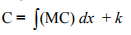where k is the constant of integration which can be evaluated if the fixed cost is known. If the fixed cost is not known, then k = 0.

Average cost fucntion,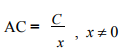Example 12
The marginal cost function of manufacturing x units of a commodity is 6 + 10x - 6x2 . Find the total cost and average cost, given that the total cost of producing 1 unit is 15.

Solution : Given that,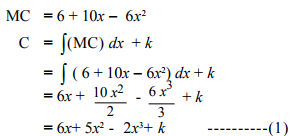Given, when x = 1,C = 15
15= 6 + 5 - 2 + k
⇒ k = 6
∴ Total Cost function, C = 6x + 5x 2- 2x 3 + 6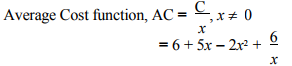Example 13
The marginal cost function of manufacturing x units of a commodity is 3x2 - 2x + 8. If there is no fixed cost find the total cost and average cost functions.

Solution : Given that,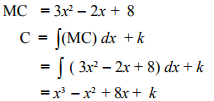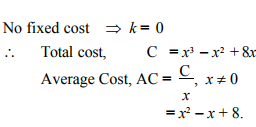Example 14 The marginal cost function of manufacturing x units of a commodity is 3 - 2x -x 2 . If the fixed cost is 200, find the total cost and average cost functions.
Solution : Given that,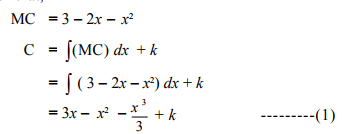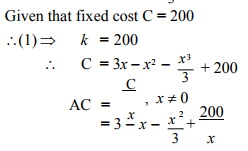The revenue function and demand function from marginal revenue function

If R is the total revenue function when the output is x, then marginal revenue MR = dx/dR . Integrating with respect to ‘x’ we get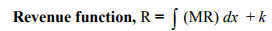where ‘k’ is the constant of integration which can be evaluated under given conditions. If the total revenue R = 0, when x = 0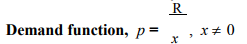Example 15 If the marginal revenue for a commodity is MR = 9 - 6x2 + 2x, find the total revenue and demand function.
Solution : Given that, MR = 9 - 6x 2 + 2x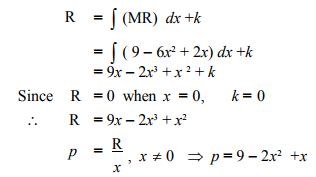Example 16
For the marginal revenue function MR = 3 - 2x - x 2 , find the revenue function and demand function.

Solution : Given that
MR = 3 - 2x - x2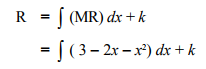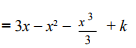since R = 0, when x = 0, k = 0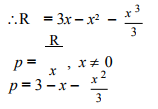Example 17
If the marginal revenue for a commodity is​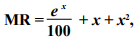find the revenue function.

Solution : Given that,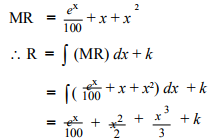when no product is sold, revenue is zero. when x = 0, R = 0.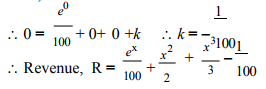The demand function when the elasticity of demand is given
We know that, Elasticity of demand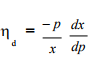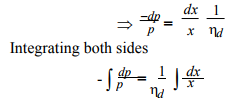This equation yields the demand function ‘ p’ as a function of ‘x ’.
The revenue function can be found out by using the relation, R = px .

Example 18
The elasticity of demand with respect to price p for a commodity is demand x- 5/x , x > 5 when the demand is ‘x’. Find the if the price is 2 when demand is 7. Also find function the revenue function​

Solution : Given that, Elasticity of demand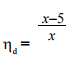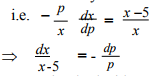Integrating both sides,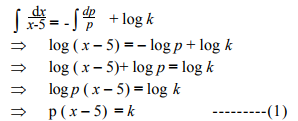when p = 2, x = 7, k = 4
∴ The demand function is,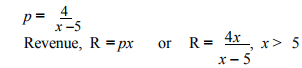Example 19
The elasticity of demand with respect to price for a commodity is a constant and is equal to 2. Find the demand function and hence the total revenue function, given that when the price is 1, the demand is 4.

Solution : Given that, Elasticity of demand, ηd = 2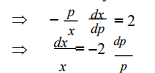Integrating both sides,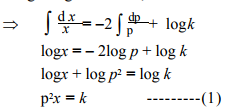Given, when x = 4, p = 1
From (1) we get k = 4
∴  (1) ⇒ xp2 = 4 or p2 = 4/x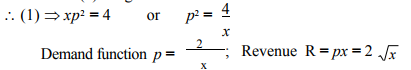Example 20
Revenue R = px = 2 x The marginal cost and marginal revenue with respect to a commodity of a firm are given by C' (x) = 4 + 0.08x and R'(x) = 12. Find the total profit, given that the total cost at zero output is zero.

Solution :
Given that,
MC = 4 + 0.08x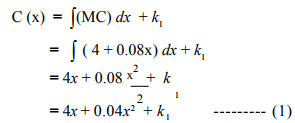But given when x = 0,C = 0
∴ (1)⇒ 0 = 0 + 0 + k
∴ k = 0
∴ C(x) = 4x + 0.04x 2 ---------(2)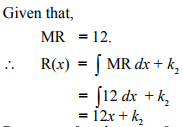Revenue = 0 when x = 0.
∴ k = 0
∴ R (x) = 12x ---------(3)
Total profit function, P(x) = R(x) - C (x)
= 12x - 4x - 0.04x2
= 8x - 0.04x2 .

Example 21 The marginal revenue function (in thousands of rupees) of a c o m mo d ity is 7 + e -0.05x where x is the number of units sold. Find the total revenue from the sale of 100 units ( e -5 = 0.0067)

Solution : Given that,
Marginal revenue, R'(x) = 7 + e - 0.05x
∴ Total revenue from sale of 100 units is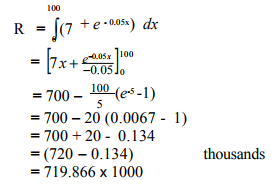Revenue, R = Rs.7,19,866.

Example 22 The marginal cost C'(x) and marginal revenue R'(x) are x given by C'(x) = 20 +x/20 and R'(x) = 30 The fixed cost is Rs. 200. Determine the maximum profit. Solution :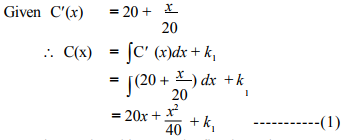When quantity produced is zero, the fixed cost is Rs. 200. i.e. when x = 0, C = 200,
⇒ k = 200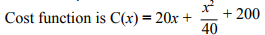The revenue, R'(x) = 30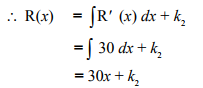When no product is sold, revenue = 0 --------(2)
i.e., when x = 0, R = 0
∴ Revenue, R(x) = 30x
Profit, P = Total revenue - Total cost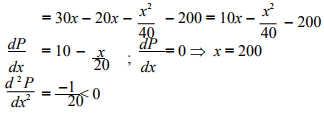∴ Profit is maximum when x = 200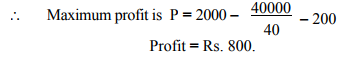Example 23 A company determines that the marginal cost of producing x units is C'(x) = 10.6x. The fixed cost is Rs. 50. The selling price per unit is Rs. 5. Find (i) Total cost function (ii) Total revenue function (iii) Profit function.

Solution : Given,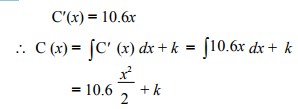= 5.3x2 + k --------(1)
Given fixed cost = Rs. 50

(i.e.) when x = 0, C = 50 ∴ k = 50
Hence Cost function, C = 5.3x 2 + 50

(ii) Total revenue = number of units sold x price per unit Let x be the number of units sold. Given that selling price per unit is Rs. 5.
∴ Revenue R(x) = 5x.
(iii) Profit, P= Total revenue - Total cost

= 5x - (5.3x 2 + 50)
= 5x - 5.3x 2 - 50.

Example 24 Determine the cost of producing 3000 units of commodity  if the marginal cost in rupees per unit is C'(x)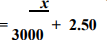Solution : Given, Marginal cost, C'(x)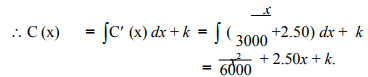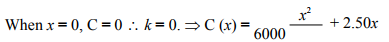When x = 3000,
Cost of production, C(x) = Rs.9000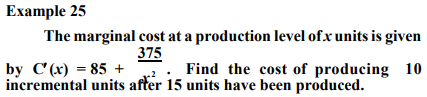Solution :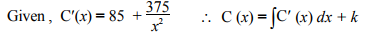The cost of producing 10 incremental units after 15 units have been produced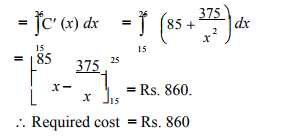Offer running on EduRev: Apply code STAYHOME200 to get INR 200 off on our premium plan EduRev Infinity!

122 videos|142 docs

,

,

,

,

,

,

,

,

,

,

,

,

,

,

,

,

,

,

,

,

,

,

,

,

;